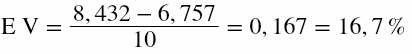## What is Expected Value for casino games

Expected Value is the long-run average value of repetitions of the experiment it represents. This we usually call simply as “Expectation” and doesn’t tell us if and how much we can win or lose by a specific bet in a gambling game.

In gambling, by Expected Value or Average Expectation, we mean the calculation of how much money we will win or lose in the long run based on the odds and payouts of the game. Some clarifications are needed:

1. The amount of money we should expect to win or lose is usually given as a percentage of the money wagered.
2. By long run we mean after many thousands of trials. The more trials the closer we will get to the expected value prediction.
3. This is a relatively accurate theoretical prediction of the long run result of our play, but still, actual results can vary.

## The House Edge of casino games

Naturally, all casino games have a negative expected value. All players are expected to lose in the long run. The games are designed in favor of the casino. Actually, the expected value of any casino games is equal (in a negative way) to the house edge – they are the same thing. Specifically here is the expected value or house edge of the main casino games:

• Roulette (double zero): -5,26%
Roulette (single zero): -2,7%
Roulette (even chance bets, single zero with La Partage or En Prison rule): -1,35%
• Blackjack: -0,5%
• Caribbean Stud Poker: -5,22%
• Craps Don’t Pass: -1,36%
Craps Come: -1,41%
Craps Tie: -14,35%
Craps Pair: -10,36%
• Baccarat Banker: -1,05%
Baccarat Player: -1,2%
• Video Poker: -1% (varies – depends on the game)
• Slot Machines: -10% (varies – depends on the game)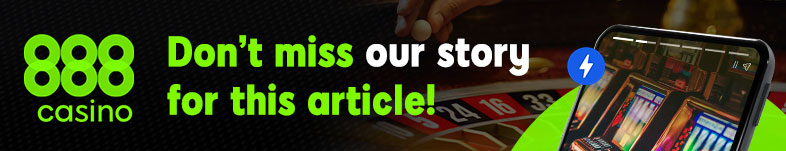This negative expected value or house edge is the one and only reason that mathematicians claim that it is impossible to win a game of chance in the long run. I have explained with arguments my disagreement to this thesis, in the house edge monograph.

## How to calculate the expected value of any game and any bet

Sometimes there can be special rules or maybe the is no fixed house edge. You can always calculate the probability and the expected value (negative house edge) of any bet by using the following equation: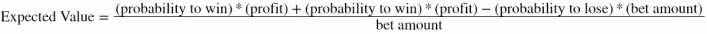If we have multiple bets at the same time: We multiply the probability of our bets to win with their respective profits in case of win and we add them together. Then we deduct the probability to lose multiplied by the loss (which is our bet amount). Then we divide the sum to the amount of our bet.

If we only make one bet, then the equation is: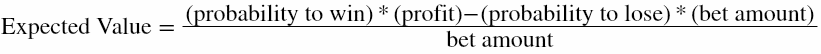Now to make things even more simple we can use as bet amount just 1 unit. Whether we bet more or less the expected value will be a fraction of our bet, so it makes things more simple to calculate if we use 1 (unit/chip/dollar/euro whatever) as our bet. In this case the equation becomes: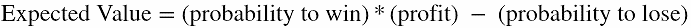### Dozen Example

Now let’s calculate the expected value of the dozen bet in roulette. We already know (see previous section) that every bet in single zero roulette has an expected value (or house edge) equal to -2,7%, but let’s calculate it ourselves.

For a dozen, the probability to win is 12/37 because we are effectively betting on 12 numbers from 37 total numbers. The profit in case of win is 2 units. The probability to lose is 25/37, because 25 numbers out of 37 make us lose. So the Expected value of the dozen bet is: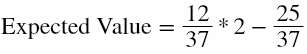Which gives: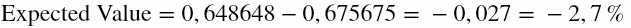Which means that “in the long run” we expect to lose an amount equal to 2,7% of the total amount wagered.

### Fantasy Example

A question from our forum:

Let’s say the that I was playing the following numbers: 1-2-3-4-5-6-7-8-9-10 and was betting 1 chip on each number. Now the croupier congratulates me for my birthday and says “you can play two extra numbers where you don’t have to pay” and I add
11-12. So basically now I am playing 12 straight numbers for the price of 10. What would be my advantage?

Let’s calculate the expected value of this fantasy bet. We are betting 12 numbers with only 10 chips and each number pays 35 units profit. We have: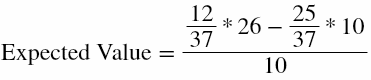Which gives: